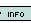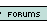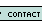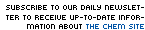1. A container contains 0.20 moles of O2 and 0.15 moles of H2. If the temperature of the container is 273 Kelvin, which is true? The average molecular speeds of the two gases are the same The total masses of the two gases are the same The partial pressures of the two gases are the same The average kinetic energy of the two gases are the same The molecular masses of the two gases are the same 2. An oxygen sample contains volume of 6.00 L at 25oC and 900. torr. How many molecules does this sample contain? 0.291 0.502 2.46*10^23 1.75*10^23 6.02*10^23 3. A mixture of gases contains 48 g O2, 84 grams N2, 9.0 grams of water vapor. If the total pressure is 700 mmHg, what is the partial pressure of the nitrogen gas? 0.092 atm 0.28 atm 0.37 atm 0.46 atm 0.55 atm 4. What is the root mean square velocity of methane (CH4) at 273oK? 1 m/s 1304 m/s 310 m/s 652 m/s 16 m/s 5. If you have a gas taking 14.0 L at 800. torr and 26oF, calculate the volume of the gas at 40oC and 2 atm. 4.13 L 16.34 L 8.27 L 5674 L 4.26 L Name: E-mail: Last 15 Challengersnews | about us | contact us tutorials index | organic chemistry | practice tests | online quizzes | reference tools site copyright (c) 2002 to neopages.com